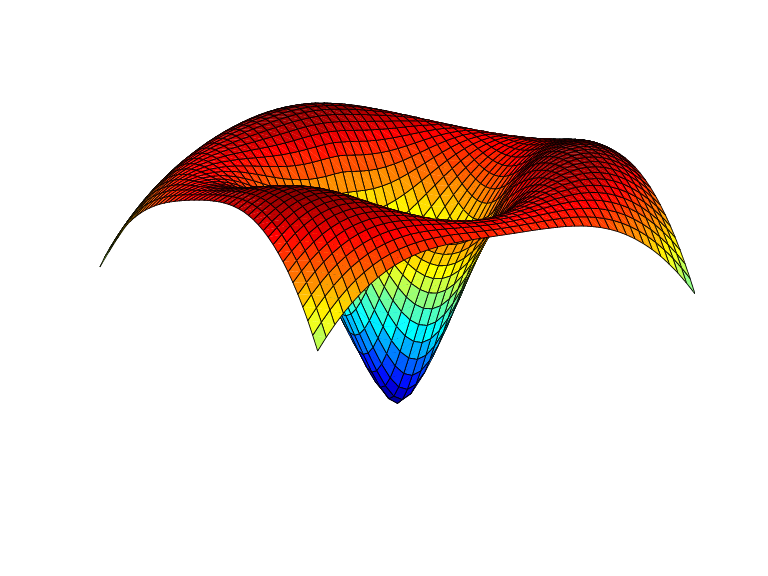# stcol

Scattered translates collocation matrix

## Syntax

```colmat = stcol(centers,x,type) colmat = stcol(...,'tr') ```

## Description

`colmat = stcol(centers,x,type) ` is the matrix whose (`i`,`j`)th entry is

with the bivariate functions ψj and the number `n` depending on the `centers` and the character vector or string scalar `type`, as detailed in the description of `stmak`.

`centers` and `x` must be matrices with the same number of rows.

The default for `type` is the character vector `'tp'`, and for this default, `n` equals `size(centers,2)`, and the functions ψj are given by

with ψ the thin-plate spline basis function

`$\psi \left(x\right)={|x|}^{2}\mathrm{log}{|x|}^{2}$`

and with |x| denoting the Euclidean norm of the vector x.

Note

See `stmak` for a description of other possible values for `type`.

The matrix `colmat` is the coefficient matrix in the linear system

that the coefficients aj of the function f = Σjajψj must satisfy in order that f interpolate the value yi at the site `x(:,i)`, all `i`.

`colmat = stcol(...,'tr') ` returns the transpose of the matrix returned by `stcol(...)`.

## Examples

Example 1. The following evaluates and plots the function

`$f\left(x\right)=\psi \left(x-{c}_{1}\right)+\psi \left(x-{c}_{2}\right)+\psi \left(x-{c}_{3}\right)-3.5\psi \left(x\right)$`

on a regular mesh, with ψ the above thin-plate basis function, and with c1, c2, c3 three points on the unit circle; see the figure below.

```a = [0,2/3*pi,4/3*pi]; centers = [cos(a), 0; sin(a), 0]; [xx,yy] = ndgrid(linspace(-2,2,45)); xy = [xx(:) yy(:)].'; coefs = [1 1 1 -3.5]; zz = reshape( coefs*stcol(centers,xy,'tr') , size(xx)); surf(xx,yy,zz), view([240,15]), axis off ```Example 2. The following also evaluates, on the same mesh, and plots the length of the gradient of the function in Example 1.

```zz = reshape( sqrt(... ([coefs,0]*stcol(centers,xy,'tp10','tr')).^2 + ... ([coefs,0]*stcol(centers,xy,'tr','tp01')).^2), size(xx)); figure, surf(xx,yy,zz), view([220,-15]), axis off ```